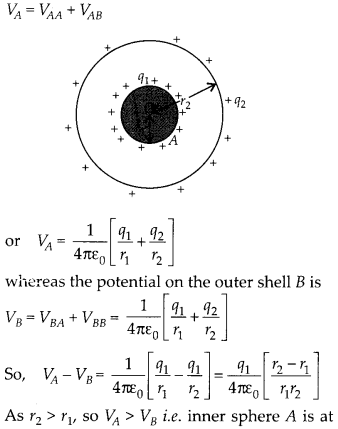Enlightened

# Question 36: NCERT Solutions for 12th Class Physics: Chapter 2-Electrostatic Potential and Capacitance

• 0

Question 36: NCERT Solutions for 12th Class Physics: Chapter 2-Electrostatic Potential and Capacitance

A small sphere of radius r1 and charge q1 is enclosed by a spherical shell of radius r2 and charge q2. Show that if q: is positive, charge will necessary flow from the sphere to the shell (when the two are connected by a wire) no matter what the charge q2 on the shell is.

Share

1. Solution:
The potential on inner small sphere is VAhigher potential than outer conducting shell B, for any value of charge qv So, when inner sphere A is connected to outer shell B, then charge will flow from inner sphere A to outer shell B, until electric potentials on them is same i.e.
VA – VB = 0 or q1 = 0 [As r1 # r2]

So, charge ql given to sphere A will flow on the shell B, no matter what the charge on the shell B is.

Check the complete chapter with solutions.

NCERT Solutions for 12th Class Physics: Chapter 2-Electrostatic Potential and Capacitance

• 0